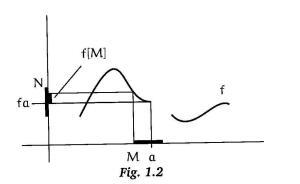# What book are good to learn about limits and continuity for the first time?

I've tried reading about "open balls", limits, and continuity, but I just don't get the stuff about " given $\epsilon > 0$ find $\delta > 0$ such that $\ldots < \delta$ implies that $\ldots < \epsilon$". I've tried to read about this in a few books but I still don't understand it. I've read about it in Spivak's Calculus and the book I'm holding now that thinks it should be "clear". Are there any books that are good for someone just beginning math beyond calculus or that just completely spell out the reasoning behind this kind of argument?

• have you tried watching series of online videos on this subject? books on calculus are usually written rigorously while video can give you more of an intuition and understanding of what are the main topics you should be aware of. – Anonymous Apr 8 '12 at 23:49

Unfortunately, I think that Spivak is a good intro book in that regard. Perhaps now you just need some examples. So I recommend that you look up or try to find some examples, and ask here if you've any questions.

And I think you'll find that many other people have had trouble with $\epsilon - \delta$ limits as well. A good source to improve your understanding of calculus-related material is at Paul's Online Math Notes (I've linked to the epsilon-delta limit page).

I'd be interested to know if you find helpful the account in my book Topology and Groupoids (T&G). Chapter $1$ gives an account of neighbourhoods on the real line, which is a more geometric version of traditional $\epsilon$-$\delta$ arguments. The point is that $\epsilon$, $\delta$ are measures of the size of a symmetric interval about $0$, and it is often easier to think of the interval itself, and how it is transformed by a function, rather than use the measure of its size, which is one step away from the geometry. You need PICTURES!

This account using neighbourhoods leads easily to more general situations, especially if the notion of limit is linked with the notion of continuity. Thge definition of this is that a function $f$ is continuous at $a$ if for all neighbourhooods $N$ of $f(a)$ there is a neighbourhood $M$ of $a$ such that $f[M] \subseteq N$:One needs to think about other situations. Consider the fact that, approximately, the volume of a radio is a continuous function of the position of the volume knob. But the output is a signal! This output is (approximately) not a continuous function of the position of the tuning knob, since one wants a sharp tuning.

The relation between limits and continuity is illustrated as follows. Suppose we want to say $\lim_{x\to a} f(x) =l$, where $f$ is not defined at $a$, for example $f(x)= (x^2-a^2)/(x-a)$. Define a new function $g$ to be $f$ when $x \ne a$ and to be $2a$ at $a$. Then the limit of $f$ at $a$ is $2a$ because $g$ is continuous at $a$.

See also the section Motion of a lecture I gave at the Royal Institution. This is relevant to Section 1.4 of T&G, on Some Generalisations.

If you don't get "the stuff about given epsilon find delta" as you say, you may want to consult Keisler's textbook Elementary Calculus. It's exactly what you are looking for because in Keisler's approach, the key concepts of the calculus like continuity and derivative are explained without "the stuff about given epsilon find delta". Keisler uses rigorous infinitesimals instead. The epsilon-delta paraphrases are introduced later, once the student has already learned the key concepts via the intuitively appealing infinitesimal definitions.

• I don't understand how this answers the question. More specifically, how does this answers OP's question: "Are there any books that are good for someone just beginning math beyond calculus or that just completely spell out the reasoning behind this kind of argument?" – user 170039 Nov 1 '17 at 13:28
• @user170039, I spelled this out in the body of my answer. – Mikhail Katz Nov 1 '17 at 14:32